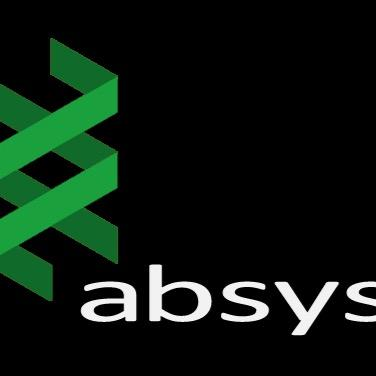# GluVarPro

March 2019### Introduction

This application calculates different glucose variability measures, including average measures of glycemia, measures of glycemic variability, and measures of glycemic risk, from continuous glucose monitoring data obtained from diabetic patients.

### Sections

The application is divided into five parts or sections:

• Data Selection.
• Data Visualization.
• Glycemic Variability Measures.
• Glycemic Variability Tables.
• Results Generation.

### Data Selection

The user can fill empty values with two different functions: linear or cubic splines; and can select the maximum interval to fill the empty values.

The application shows the number of observations included in the data, the number of days, the range of glucose values and the number of empty values.

### Data Visualization

The application represents the data as time series where the X axis represents the time and the Y axis the glycemia values in mg/dl.

The user can select the color for plotting data points. If the data-set contains more than one day, different colors can be selected for each day of data.

### Glycemic Variability Measures

The application calculates the following average measures of glycemia:

• MEAN (Arithmetic mean).
• AUC (Area Under Curve).
• LAUC (Low Area Under Curve).
• HAUC (High Area Under Curve).
• PSTR (Percentage Spent below/above the Target Range).
• LPSTR (Low Percentage Spent below the Target Range).
• HPSTR (High Percentage Spent above the Target Range).
• NPSTR (Normal Percentage Spent between the Target Range).

### Glycemic Variability Measures

The application calculates the following glycemic variability measures:

• ADRR (Average Daily Risk Range).
• BGI (Blood Glucose Index).
• LBGI (Low Blood Glucose Index).
• HBGI (High Blood Glucose Index).
• CONGA (Continuous Overall Net Glycemic Action).
• CV (Coefficient of percentual Variation).
• IQR (InterQuartile Range).
• JI (J Index).

### Glycemic Variability Measures

• LI (Lability Index).
• MAGE (Mean Amplitude of Glycemic Excursions).
• GE (Glycemic Excursions).
• LMAGE (Low Mean Amplitude of Glycemic Excursions).
• HMAGE (High Mean Amplitude of Glycemic Excursions).
• MV (M Value).
• SD (Standard Deviation).

### Glycemic Variability Measures

The user can select the interval to calculate the measures and the sampling time of the data.

The measures are represented by graphs in each tab panel. If data-set contains more than one day, different colors can be selected for each day of data.

### Glycemic Variability Measures

The user can select different parameters to evaluate the measures:

• Low Target Range (for measures AUC and PSTR).
• High Target Range (for measures AUC and PSTR).
• Number of hours (for measure CONGA).
• Multiples of sd (for measure MAGE).
• Ideal glucose (for measure MV).

### Glycemic Variability Tables

The application shows two different tables. The first one is a table with variability measures for each data point. The second one shows the mean values of the measures for each day along with four different deviations:

• sd (standard deviation).
• sem (standard error of the mean).
• rmse (root mean square error).
• mase (mean absolute scale error).

### Results Generation

The application shows the analysis of results based on the mean values of the measures for each day.

The application can generate a pdf file with the results of the mean values of the different glycemic variability measures, along with the deviations, plus the parameters selected by the user.

In addition, it allows the generation of two csv files with the same data that appears in the Glycemic Variability Tables section.

We recommend you try all these features here!!